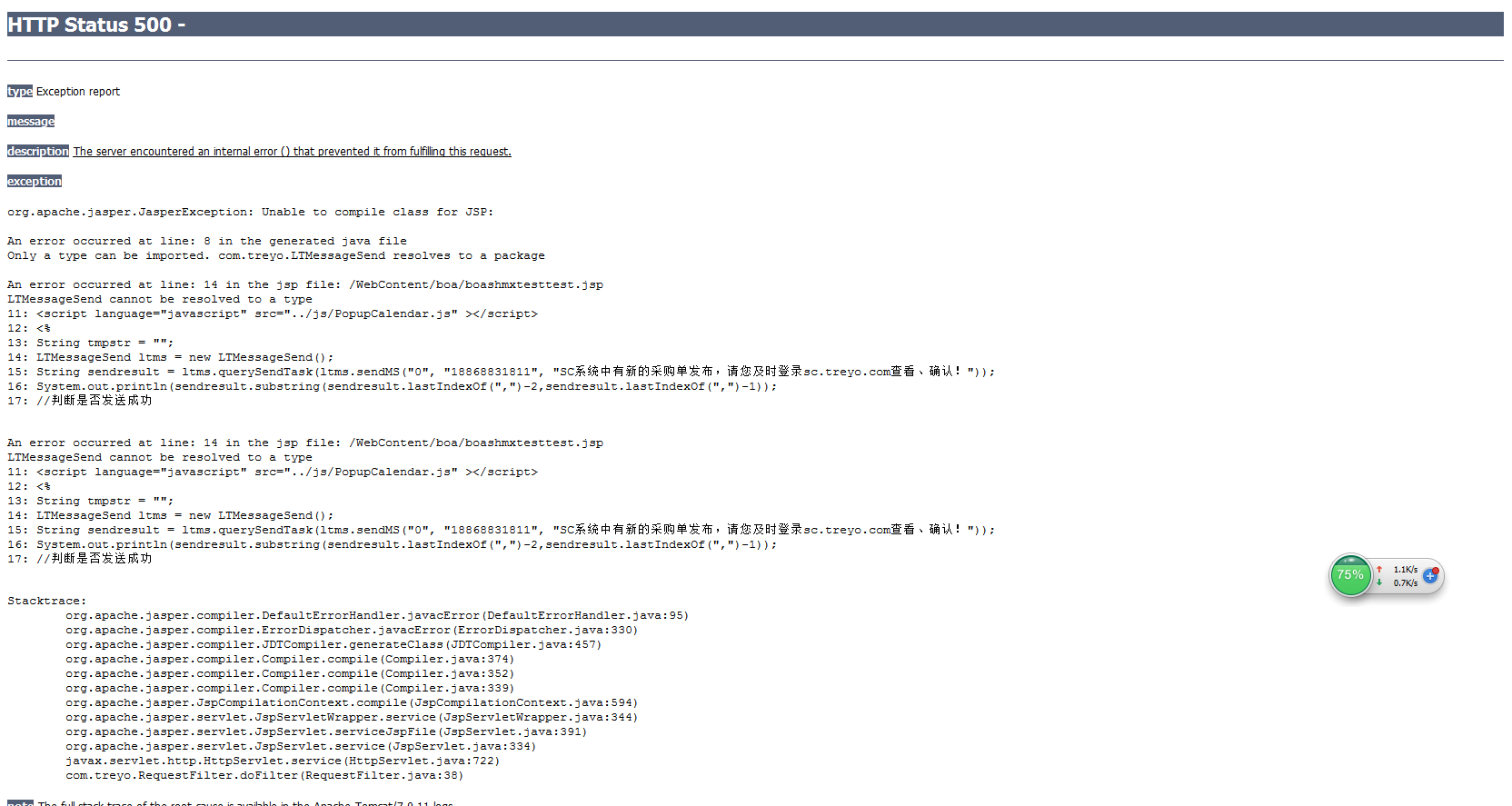<%
String tmpstr = "";
LTMessageSend ltms = new LTMessageSend();
String sendresult = ltms.querySendTask(ltms.sendMS("0", "188xxxxxxxx", "SC系统中有新的采购单发布，请您及时登录sc.treyo.com查看、确认！"));
System.out.println(sendresult.substring(sendresult.lastIndexOf(",")-2,sendresult.lastIndexOf(",")-1));
//判断是否发送成功
if(sendresult.substring(sendresult.lastIndexOf(",")-2,sendresult.lastIndexOf(",")-1)=="4"){
tmpstr = tmpstr + "短信发送成功！";
}
else {
tmpstr = tmpstr + "短信发送失败，请联系管理员！";
}
// 短信保存
//20170510修改结束-----
%>

test

<<<<<<<<<<<<<<<<<<<<<<<<<<<<<<<<<<<<<<<<<<<<<<<<<<<<<<<<<<<<<<<<<<<<<<<<<<<1个回答